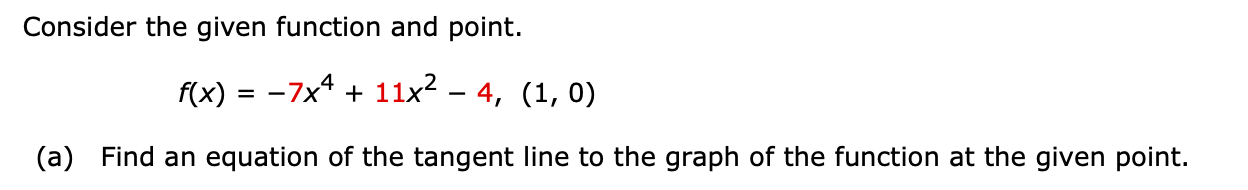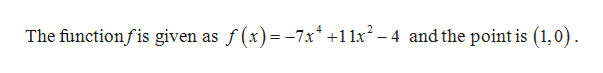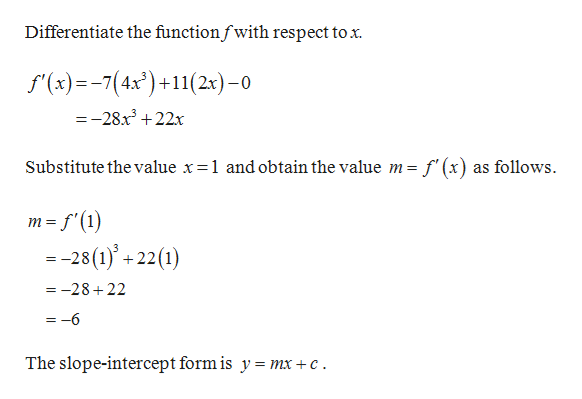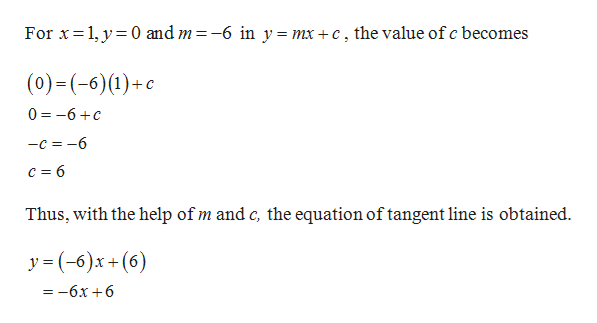Consider the given function and point.f(x) = -7x4 11x2 - 4, (1, 0)(a) Find an equation of the tangent line to the graph of the function at the given point.

Question

Hi, I am having a hard time figuring out what the equation of the tangent line is in this problem. When I did it I got y=-8x+8 but it's wrong. If someone could help me figure how to get the right answer that would be very helpful!help_outlineImage TranscriptioncloseConsider the given function and point. f(x) = -7x4 11x2 - 4, (1, 0) (a) Find an equation of the tangent line to the graph of the function at the given point. fullscreen
Step 1

Given:help_outlineImage TranscriptioncloseThe functionfis given as f(x)-7x 12-4andthe point is (1,0) fullscreen
Step 2

Calculation:help_outlineImage TranscriptioncloseDifferentiate the functionfwith respect tox f(x)7(4x)11(2x)-0 =-28x322x f'(x) as follows Substitute thevalue x=1 and obtain the value m = m f(1 = -28(1)'+ 22(1) =-28+22 = -6 The slope-intercept form is y = mx +c fullscreen
Step 3

Substitute the ...help_outlineImage TranscriptioncloseFor x 1,y 0 and m = -6 in y = mx +c, the value of c becomes (0) - (-6) (1)+с 0 -6c —с %3D —6 c 6 Thus, with the help of m and c, the equation of tangent line is obtained y (6)x+(6) =-6x +6 fullscreen

Want to see the full answer?

See Solution

Want to see this answer and more?

Our solutions are written by experts, many with advanced degrees, and available 24/7

See Solution
Tagged in

Derivative